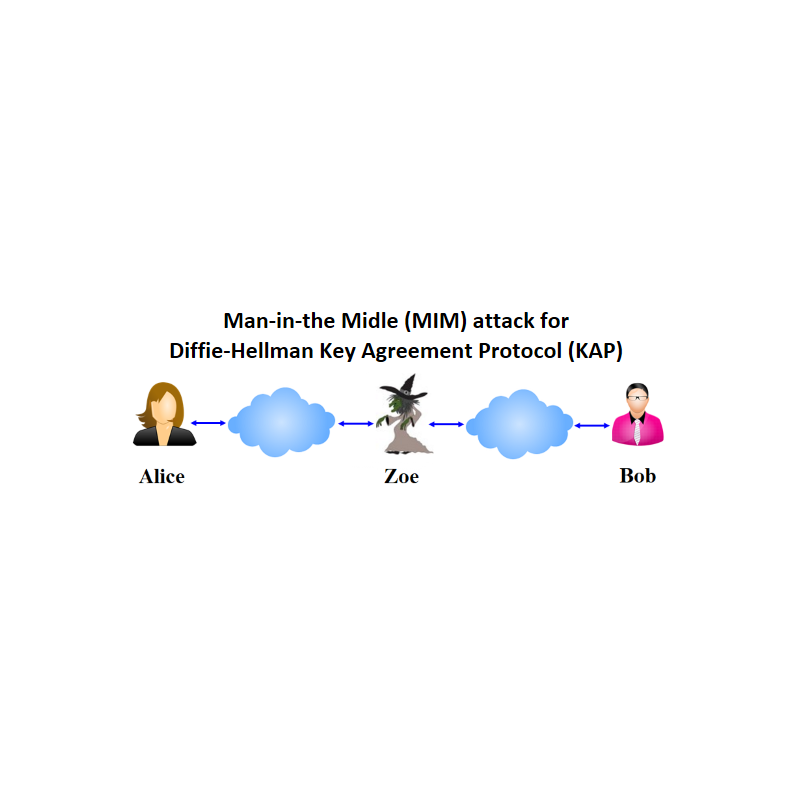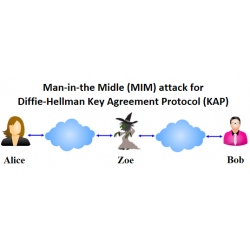•Man-in-the-Middle Attack

€4.00
Tax included

Man-in-the-Middle (MIM) attack to Diffie-Hellman (DH) Key Agreement Protocol (KAP).

Quantity

COMPLETED.

Imagine You are an active eavesdropper Zoe and want to arrange Man-in-the-Middle (MIM) attack to Diffie-Hellman (DH) Key Agreement Protocol (KAP) between Alice and Bob which actions are simulated by the Mentor. Parameter values sent by Zoe to Mentor are entered in the input field and are included in brackets [ ] and must be entered in the corresponding input fields.

The following functions are used in the protocol:

>> mod_exp(g,x,p)

>> randi(z)

System parameters known for all parties are prime number p=147146159 and generator g=17, where p is 28 bits length strong prime. Please check it for awareness. Then MIMDH attack is performed in the following way.

1. Alice randomly generates secret number x in the interval 1<x<p-1. She computes public parameter KA=gx mod p and sends KA=….. to Bob. An active eavesdropper Zoe intercepts KA and stops message transmission to Bob. Zoe impersonates Bob by randomly generating secret number z1 in the interval 1<z1<p-1, using function randi(2^25), computes public parameter KAZ=gz1 mod p and sends this number [KAZ] to Alice.

2. Analogously, to impersonate Alice, Zoe randomly generates secret key z2 in the interval 1<z2<p-1, computes public parameter KZB=gz2 mod p and sends [KZB] to Bob.

3. Alice presuming that message containing KAZ is received from Bob, computes the agreed secret key K1=( KAZ)x mod p.

4. Bob presuming that message containing KZB is received from Alice, randomly generates secret number y in the interval 1<y<p-1. He computes public parameter KB=gy mod p and sends KB to Alice. Then Bob computes the agreed secret key K2=(KZB)y mod p, thinking that Alice has the same secret key. But Zoe intercepts KB and stops message transmission to Alice.

5. Zoe computes secret keys KZ1=(KA)z1 mod p and KZ2=(KB)z2 mod p. Using modular mathematics she proves to herself that KZ1= K1 and KZ2= K2.

6. What does it means? How Zoe knows the content of all correspondence during the session?

7.      You can test Yourself in arranging MIMDH attack with the following artificially small public parameters: p=31 and g=11 using local computations with Octave. If the intercepted message from Alice-to-Bob is KA=13 and intercepted message from Bob-to-Alice is KB=18, then the following sequence would be valid for the protocol: z1=3, z2=4, KAZ=9, KZB=29, KZ1=10, KZ2=4.

3104 Items